f
Neutrium

# Welcome to Neutrium

Neutrium is a knowledge base of engineering topics, centred mainly around chemical engineering design challenges faced by engineers in their daily work. We created Neutrium to bridge the gap between theory and practice. Feel free to ask a question, leave feedback or take a look at one of our in-depth articles.

Erosion Velocity for Gas-Liquid Multiphase Flow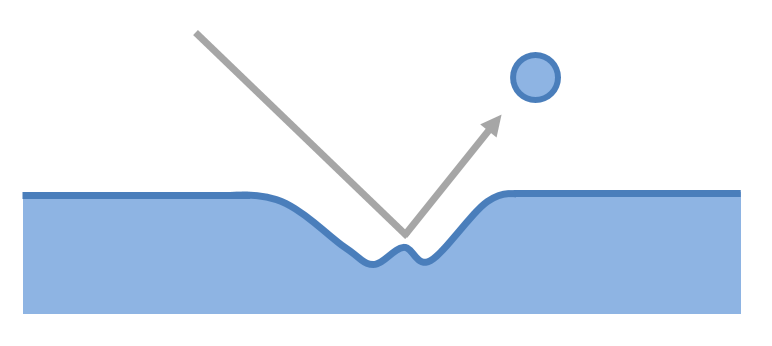The flow of a gas-liquid multiphase system may cause erosion if velocities are high. This article presents an empirical relationship for estimating whether erosion will occur in a system at a certain velocity.

Discharge Coefficient for Nozzles and Orifices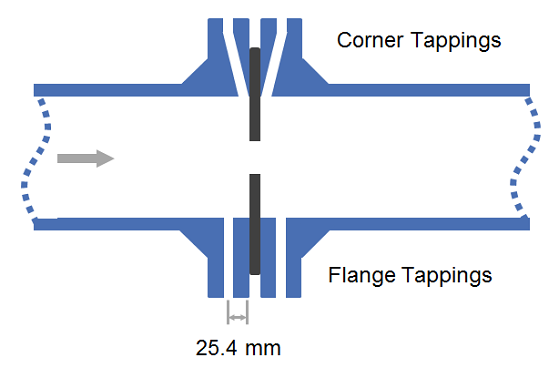The discharge coefficient is a dimensionless number used to characterise the flow and pressure loss behaviour of nozzles and orifices in fluid systems. Orifices and nozzles are typically used to deliberately reduce pressure, restrict flow or to measure flow rate. This article gives typical values of the discharge coefficient for common orifice and nozzle designs.

Calculation of Flow through Nozzles and Orifices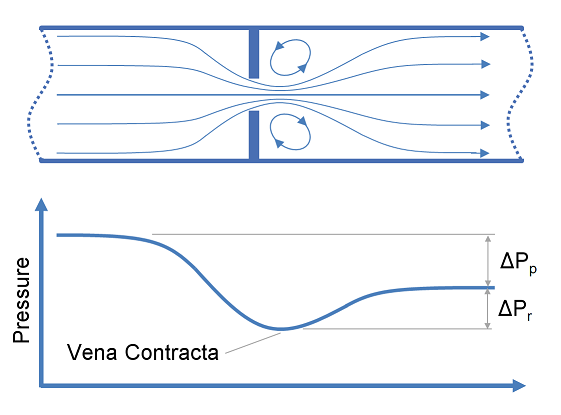This article provides calculation methods for correlating design, flow rate and pressure loss as a fluid passes through a nozzle or orifice. Nozzles and orifices are often used to deliberately reduce pressure, restrict flow or to measure flow rate.

Fitting of a Polynomial using Least Squares Method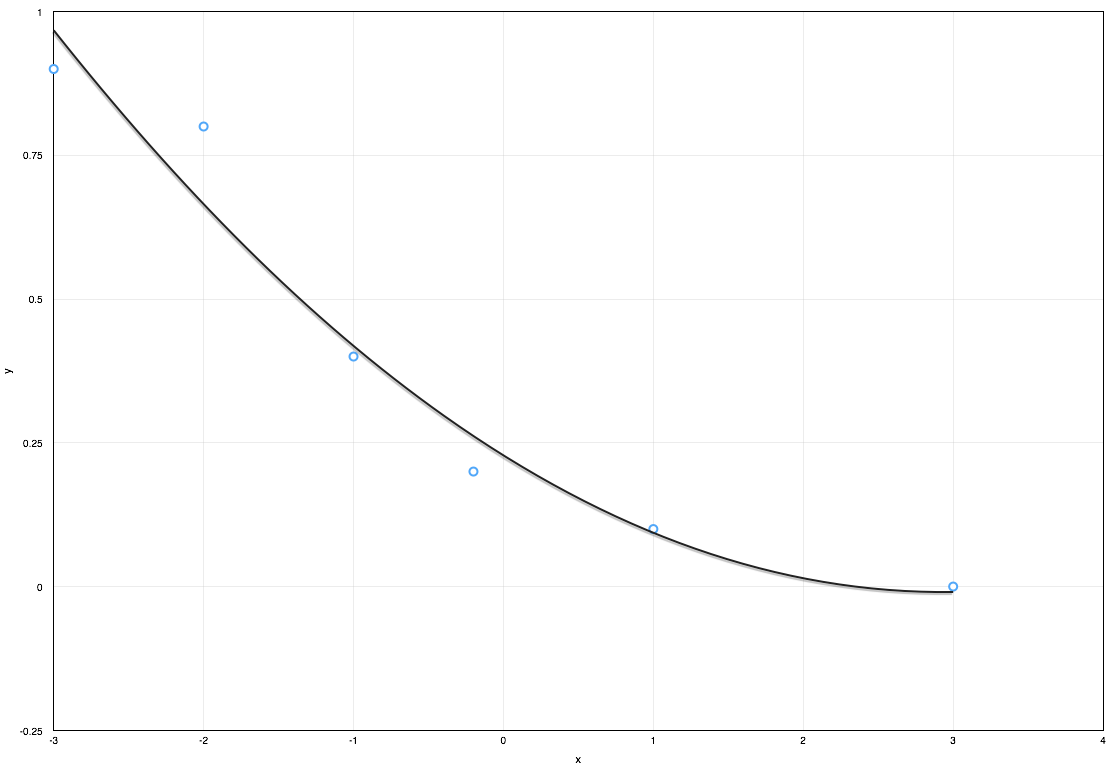Approximating a dataset using a polynomial equation is useful when conducting engineering calculations as it allows results to be quickly updated when inputs change without the need for manual lookup of the dataset. The most common method to generate a polynomial equation from a given data set is the least squares method. This article demonstrates how to generate a polynomial curve fit using the least squares method.

Joukowsky Equation

The Joukowsky equation is a method of determining the surge pressures that will be experienced in a fluid piping system. When a fluid in motion is forced to either stop or change direction suddenly a pressure wave will be generated and propagated through the fluid. This pressure wave is commonly referred to as fluid hammer (also known as water hammer, surge or hydraulic shock) and typically occurs in piping systems when a valve is suddenly closed, isolating the line. The resultant surge pressures are complex to characterise but for simple systems they may be calculated using the Joukowsky equation.

Volume and Wetted Area of Partially Filled Vertical Vessels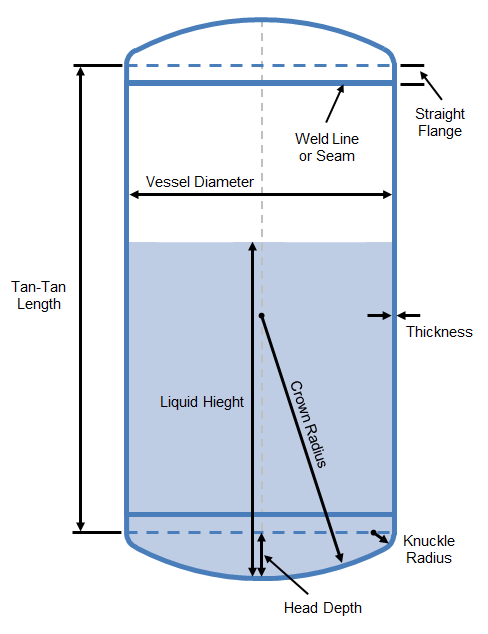The calculation of the wetted area and volume of a vertical vessel is required for engineering tasks such fire studies and the determination of level alarms and control set points. However the calculation of these parameters is complicated by the geometry of the vessel, particularly the heads. This article details formulae for calculating the wetted area and volume of these vessels for various types of curved ends including: hemispherical, torispherical, semi-ellipsoidal and bumped ends.

Manning Characteristic RoughnessThe Manning Characteristic Roughness is used to characterise the surfaces over which water can flow in streams, channels, ditches and flumes. This article presents a reference of roughness values for many common materials of construction for channels and natural formations of streams.

Flow in Open Channels and Partially Filled Pipes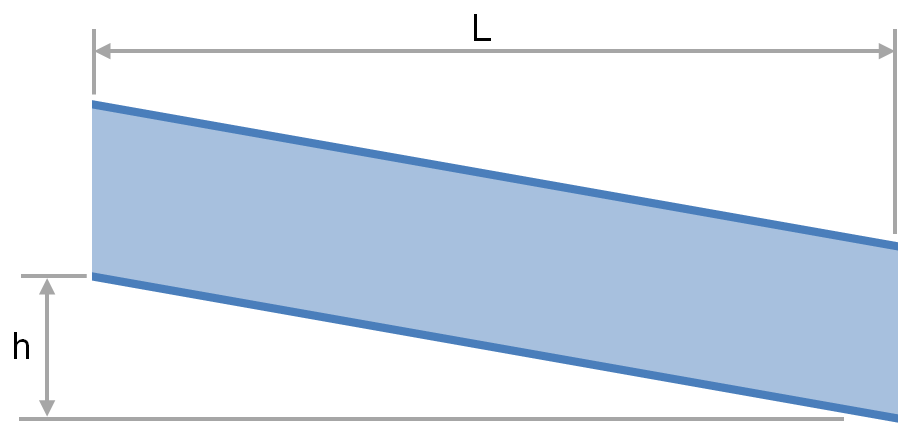The transport of fluid under gravity is often achieved using partially filled pipes, channels, flumes, ditches and streams. To determine the slope and elevation change required or the flow rate that is achievable one must be able to calculate the head loss and friction factor. This article provides relationships for the calculation of head loss and friction factor for fluids flowing via these conduits.

Mach Number

The Mach number is the ratio of the relative velocity of a fluid to the local speed of sound. This article provides the equation for the calculation of the Mach number and a discussion of its uses.

Speed of Sound in Fluids and Fluid in Pipes

This article provides the formulae for the calculation of speed of sound in fluids and fluid filled circular pipes. The speed of sounds is important in piping systems for the calculation of choked flow for gases and pressure transient analysis of liquid filled systems.